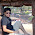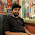### hat tip: join two spark dataframe on multiple columns (pyspark)

Consider the following two spark dataframes:
``````df1.show()
+----+------+-------+
|id_a|time_a|value_a|
+----+------+-------+
|   1|     1|     CA|
|   1|     2|     CA|
|   2|     1|     TX|
|   3|     5|     NE|
|   4|     6|     WA|
+----+------+-------+
``````
``````df2.show()
+----+------+-----------+
|id_b|time_b|    value_b|
+----+------+-----------+
|   1|     1|   San Jose|
|   2|     1|Los Angeles|
|   2|     2|     Austin|
+----+------+-----------+
``````
Now assume, you want to join the two dataframe using both `id` columns and `time` columns. This can easily be done in pyspark:
``df = df1.join(df2,(df1.id==df2.id_b)&(df1.time==df2.time),joinType="inner")``
``````df.show()
+----+------+-------+----+------+-----------+
|id_a|time_a|value_a|id_b|time_b|    value_b|
+----+------+-------+----+------+-----------+
|   1|     1|     CA|   1|     1|   San Jose|
|   2|     1|     TX|   2|     1|Los Angeles|
+----+------+-------+----+------+-----------+
``````
Note that parentheses around the conditions is absolutely necessary.

#### 8 comments:

1.How to do this in Java?

2.a.col("x").equalTo(b.col("x")).and(a.col("y").equalTo(b.col("y"))

3.how to do it in Spark 2.0 using Dataset?

4.is there a way to do this in scala

5.Can we add number of days to a date field in join condition?
Eg: df = df1.join(df2,(df1.date==df2.date+5days),joinType="inner")

6.what if we want join data on time (a new variable data) on a new datafrale

7.How to join with OR condition?

8.How to join two rdd on the basis of two keys?

## Favorite Quotes

"I have never thought of writing for reputation and honor. What I have in my heart must out; that is the reason why I compose." --Beethoven

"All models are wrong, but some are useful." --George Box

Copyright © 2015 • Ensemble Blogging